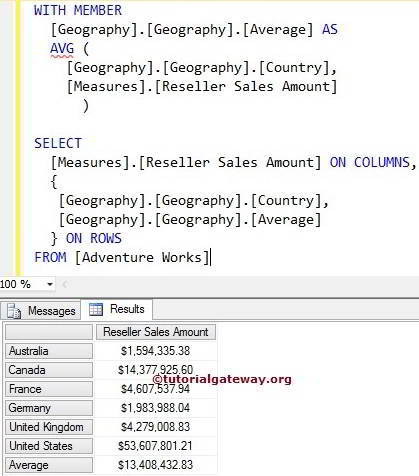# MDX AVG Function

The MDX AVG Function is used to calculate the average of non-empty members present in the specified Set. It is similar to the SQL AVG Function. The MDX Average Function will ignore the NULL values while calculating the Average

## MDX AVG Function Syntax

The basic syntax of the AVG Function in Multidimensional Expression is as shown below:

`AVG ( Set_Expression, Numeric_Expression)`
• Set_Expression: Any Multidimensional Expression that returns valid Set.
• Numeric_Expression: Any Multidimensional Expression that returns a numeric value. In general, we use the required Measure value here.

How to calculate the average using AVG function with examples?. For this, we are going to use the below-shown data.

Following screenshot shows the Countries inside the Geography

## MDX AVG Function Example 1

The following query will return the Reseller Sales amount of all the countries present in the Countries and also calculate the average of their Sales Amounts using the MDX AVG Function.

```WITH MEMBER
[Geography].[Geography].[Average] AS
AVG (
[Geography].[Geography].[Country],
[Measures].[Reseller Sales Amount]
)

SELECT
[Measures].[Reseller Sales Amount] ON COLUMNS,
{
[Geography].[Geography].[Country],
[Geography].[Geography].[Average]
} ON ROWSBelow MDX statement will create the calculated Member [Geography].[Geography].[Average]

```WITH MEMBER
[Geography].[Geography].[Average] ```

In the next line, We used the AVG Function to calculate the Average of [Measures].[Reseller Sales Amount] for the countries present in [Geography].[Geography].[Country]

```AVG (
[Geography].[Geography].[Country],
[Measures].[Reseller Sales Amount]
)```

In the next statement, We selected the Reseller Sales Amount on columns and then Set of countries and calculated Member on rows

```SELECT
[Measures].[Reseller Sales Amount] ON COLUMNS,
{
[Geography].[Geography].[Country],
[Geography].[Geography].[Average]
} ON ROWS
```

## MDX AVG Function Example 2

The following query will return the Internet Sales amount of all the calendar years present in the Date dimension. And also calculate the average of their Sales Amounts using the MDX AVG Function.

```WITH MEMBER [Date].[Calendar].[Average] AS
AVG (
[Date].[Calendar].[Calendar Year],
[Measures].[Internet Sales Amount]
)

SELECT [Measures].[Internet Sales Amount] ON COLUMNS,
NON EMPTY {
[Date].[Calendar].[Calendar Year],
[Date].[Calendar].[Average]
} ON ROWS

Below statement will create the calculated Member [Date].[Calendar].[Average]

```WITH MEMBER
[Date].[Calendar].[Average]  ```

In the next line, We used the AVG Function to calculate the Average of [Measures].[Internet Sales Amount] for the calendar years present in [Date].[Calendar].[Calendar Year]

```AVG (
[Date].[Calendar].[Calendar Year],
[Measures].[Internet Sales Amount]
) ```

In the next statement, We selected the Internet Sales Amount on columns and then Set of calendar Years and calculated Member ([Date].[Calendar].[Average]) on rows. We also used the NON EMPTY Function to avoid the NULL Values.

```SELECT [Measures].[Internet Sales Amount] ON COLUMNS,
NON EMPTY {
[Date].[Calendar].[Calendar Year],
[Date].[Calendar].[Average]
} ON ROWS

## MDX AVG Function Example 3

In this example, we will show you what happens when we have null values. For this, We are using the above MDX Query without NON EMPTY Function.

```WITH MEMBER [Date].[Calendar].[Average] AS
AVG (
[Date].[Calendar].[Calendar Year],
[Measures].[Internet Sales Amount]
)

SELECT [Measures].[Internet Sales Amount] ON COLUMNS,
{
[Date].[Calendar].[Calendar Year],
[Date].[Calendar].[Average]
} ON ROWS